# C++ .NET Functions 3

The following are the topics available in this part.

4. # Quick Reference

Stepping Through the Application with the Debugger

In this exercise, you will step through the application with the debugger. Doing so will help you understand how the flow of control passes from one function to another in your application. This exercise will also illustrate the concept of variable scope. You will see how local variables in a function come into scope during the function’s execution and disappear from scope at the end of the function.

1. Open the project from the previous exercise.

2. Locate the main() function.

3. Insert a debug breakpoint by clicking in the gray border to the left of the code. Click next to the DisplayWelcome function call, and you should see a red dot appear in the border, as shown in the following graphic.1. Start the debugging session by pressing F5. Once the program has loaded, it will execute and stop at the breakpoint in the main() function.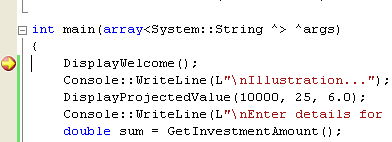A yellow arrow appears in the margin next to the DisplayWelcome function call. The yellow arrow indicates that this is the next statement to be executed.

1. Press F11 to step into the DisplayWelcome function. This debugger calls the DisplayWelcome function and displays a yellow arrow at the start of that function.1. You can also use the Debug toolbar to control the debugger. You can display the Debug toolbar by selecting Toolbars from the View menu and then choosing Debug from the list of toolbars. Each of the debug function keys mentioned in the remainder of this exercise has an equivalent Debug toolbar button.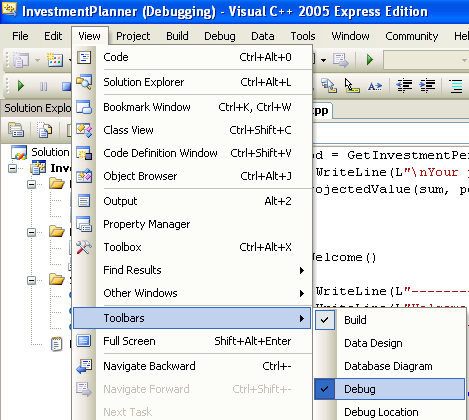1. Press F10 several times to step over each statement one at a time in the DisplayWelcome function, which will cause a welcome message to be displayed in the console window. At the end of the function, the debugger will return you to the main() function. The yellow arrow indicates the next statement to execute in main().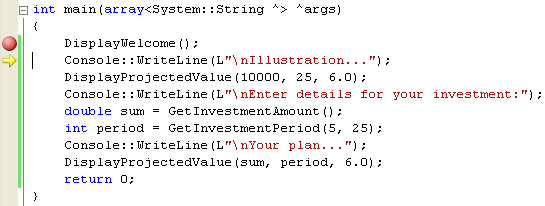1. Press F10 to step over the Console::WriteLine function. The debugger executes the Console::WriteLine function but doesn’t take you through it step by step. The yellow arrow moves on to the DisplayProjectedValue function call in main().

2. Press F11 to step into the DisplayProjectedValue function. From the Debug menu, select Windows and then Locals. The local variables in this function will be displayed.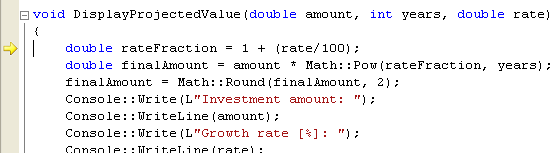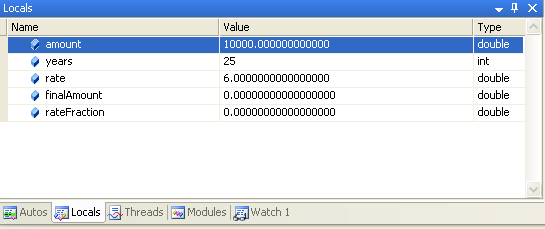The Locals window displays five local variables. The first three variables, amount, years, and rate, are the function parameters. These variables are already initialized with the values you passed into the function. The last two variables, finalAmount and rateFraction, do not have meaningful values because the variables haven’t been assigned a value yet. In fact, the debugger is a little misleading here because the finalAmount and rateFraction variables haven’t been declared yet. These variables don’t really exist until the variable declaration statements further on in the function.

1. Press F10 several times to step over the statements in the DisplayProjectedValue function. Observe how the finalAmount and rateFraction variables change during the function. The debugger displays values that were changed during the execution of the previous statement in red for prominence. Take a look at the console window to see what is displayed.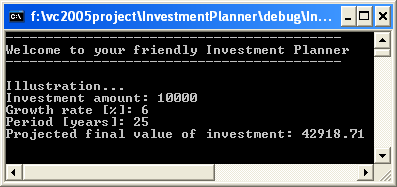1. Keep pressing F10 until you reach the end of the DisplayProjectedValue function, and return to main().

2. In main(), press F10 to step over the Console::WriteLine statement.

3. Press F11 to step into the GetInvestmentAmount function. Step through the statements in this function. When the debugger executes the ReadLine statement, the console window appears and you are asked to enter a number. Enter a number such as 20, and press Enter.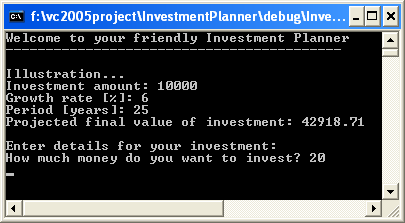1. Keep stepping through the GetInvestmentAmount function until you return to main().

2. Press F10 one more time, and then examine the local variables in main(). Notice that the return value from GetInvestmentAmount has been assigned to the sum local variable in main().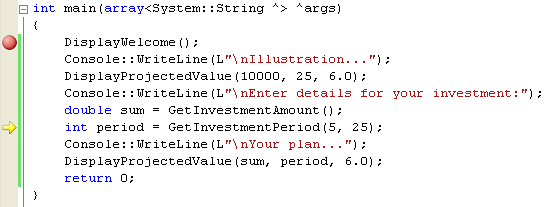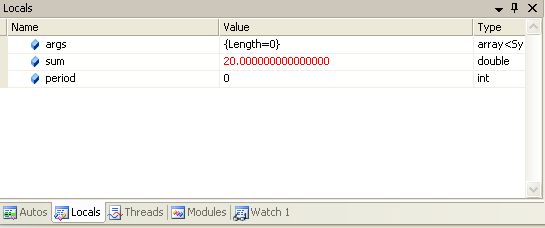1. Continue stepping through the application in this manner until the application terminates.

If the debugger takes you into a function that you’re not interested in stepping through, press Shift+F11 to step out of the function. If you just want to run the application without stopping at all, press F5.

Understanding Local and Global Scope

As you saw in the previous exercise, each function defines its own scope for local variables. The local variables are created during function execution and are automatically destroyed at the end of the function, which means you can quite happily have variables with the same name in different functions without interference. It’s also possible to declare variables globally, outside of any function. Global variables are visible in all function bodies that come after the global variable definition in your source file. You can use global variables as a rudimentary way of sharing information between multiple functions. Global variables are generally considered bad programming practice, especially in object-oriented languages such as C++. Global variables have too much visibility. Because global variables can often be used in several functions, if one gets corrupted, it can be difficult to pinpoint where the problem occurred. Global variables also introduce too much dependency between functions. For these reasons, you should use global variables sparingly. A better way of sharing information between functions is to pass parameters and return values, as you saw earlier in this module. In this exercise, you will define a global variable in your application. You will use this global variable in several functions to illustrate its global scope.

1. Continue working with the project from the previous exercise.

2. Before the start of the main() function, define a global integer variable named numberOfYourFunctionsCalled, as follows:

int numberOfYourFunctionsCalled = 0;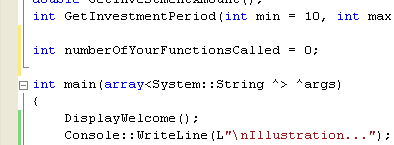1. Find the DisplayWelcome function in your code. At the start of this function, increment the numberOfYourFunctionsCalled variable as shown in the following graphic.

numberOfYourFunctionsCalled++;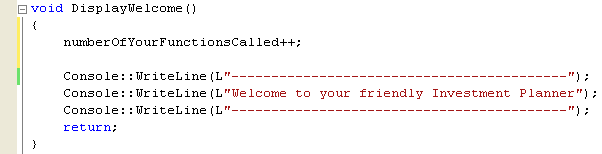1. Add a similar statement to the start of every function in your application.

2. Next, modify the main() function. At the end of this function, just before the return statement, display the value of the numberOfYourFunctionsCalled variable.

Console::Write(L"\nNumber of your functions called: ");

Console::WriteLine(numberOfYourFunctionsCalled);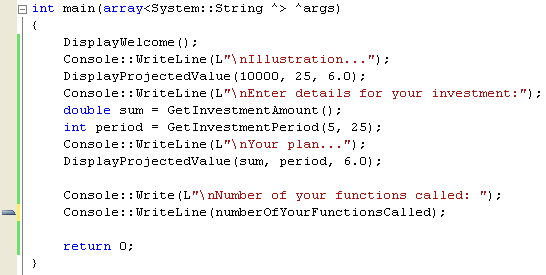1. Build and run your program. How many of your functions are called during the program?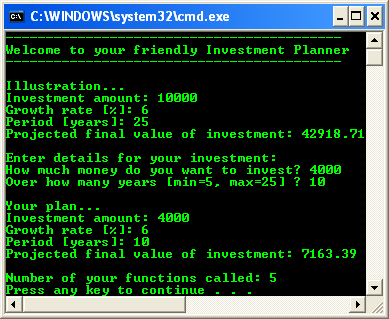Visual C++ lets you provide many functions with the same name, as long as each function has a different parameter list. This process is known as function overloading. Function overloading is useful if you have several different ways of performing a particular operation based on different input parameters. For example, you might want to provide an average function to find the average value of two double values, and you might have another average function to find the average value of an array of integers. You can define two functions to support these requirements. Give each function the same name, average, to emphasize the common purpose of these functions. Define different parameter lists for the functions to differentiate one from another.

double average(double number1, double number2);

double average(int array[], int arraySize);

You must still implement both of these functions, there is no magic here! When you call the average function, the compiler deduces which version of the function to call based on the parameter values you supply. If you define overloaded functions, the functions must have different parameter lists. If you define overloaded functions that differ only in their return type, you’ll get a compiler error.

In the following exercise, you will define an overloaded version of the DisplayProjectedValue function. The new version will calculate a random growth rate between 0 and 20 percent, rather than use a specific growth rate.

1. Continue working with the project from the previous exercise.

2. Add the following function prototype at the start of your code, near the other function prototypes:

void DisplayProjectedValue(double amount, int years);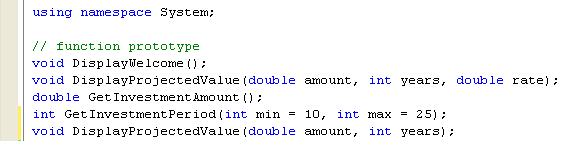1. In the main() function, locate the second call to the DisplayProjectedValue function. Modify the function call so that you pass only two parameters into the function.

DisplayProjectedValue(sum, period);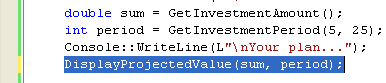1. At the end of the program, define the new DisplayProjectedValue function body as follows:

void DisplayProjectedValue(double amount, int years)

{

numberOfYourFunctionsCalled++;

Random ^ r = gcnew Random();

int randomRate = r->Next(0, 20);

DisplayProjectedValue(amount, years, randomRate);

}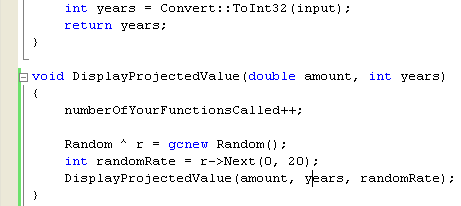This function uses the Random class to calculate a random number between 0 and 20. The function passes the random number into the original version of the DisplayProjectedValue function to calculate the value of the investment using this random rate.

1. Define breakpoints at the start of both of the DisplayProjectedValue functions.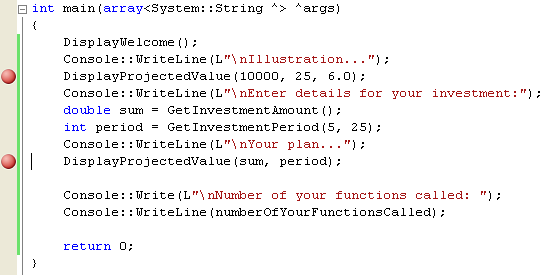1. Build the program, and start it in the debugger.

2. Observe which versions of DisplayProjectedValue are called as your program executes. See what random number the program uses for your growth rate.

3. Run the program several times to verify that the growth rate really is random.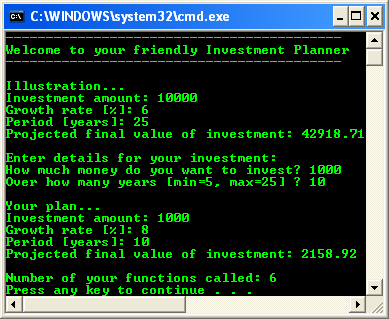Quick Reference

 To Do this Declare a function prototype. Specify the return type of the function, followed by the function name, followed by the parameter list enclosed in parentheses. Remember the semicolon at the end of the function prototype. For example:   double MyFunction(int p1, short p2); Define default parameters. Define default parameters in the function prototype, if required. Use an = operator, followed by the default value. For example:   double MyFunction(int p1, short p2=100); Define a function body. Specify the return type of the function, followed by the function name, followed by the parameter list enclosed in parentheses. Do not specify default parameters here. Define the function body within braces. For example:   double MyFunction(int p1, short p2) {     int n = p1 + p2;     // ... } Return a value from a function. Use the return keyword, followed by the value you want to return. For example:   return (p1 + p2) / 2.00; Call a function. Specify the function name, and pass parameter values within parentheses. If the function returns a value, you can assign it to a variable. For example:   double result = MyFunction(100, 175); Define and use global variables. Define the global variable outside of any function. Use the variable in any subsequent function in the source file. For example:   int myGlobal = 0;   void MyFunction() {       myGlobal++;       // ... } Define and use overloaded functions. Define several functions with the same name but different parameter lists. Implement each function. Call the version you want, using appropriate parameter values. For example:   // Prototypes void MyFunction(int p1); void MyFunction(double p1, double p2); // ... // Function calls MyFunction(100); MyFunction(2.5, 7.5); // ... // Function bodies void MyFunction(int p1) {       // ... } void MyFunction(double p1, double p2) {       // ... } Table 1